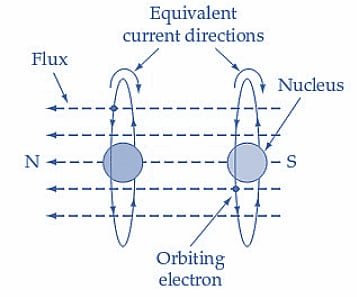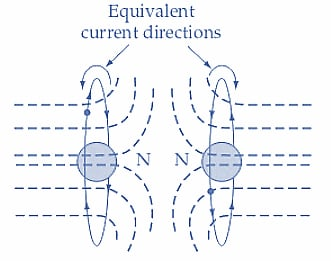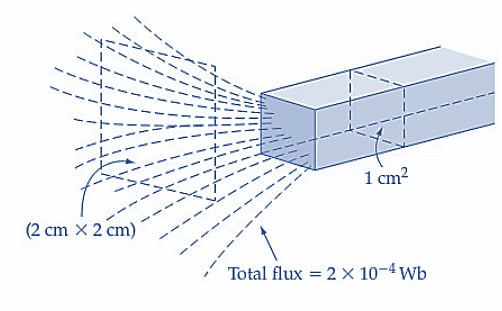Technical Article

# The Theory of Magnetism Explained

February 13, 2023 by Amna Ahmad

## This article focuses on various magnetic materials, their magnetic moments, and magnetic flux and flux density.

In relation to magnetism, all materials can be categorized into four groups.

Nonmagnetic materials have no more effect on a magnetic field than air or vacuum. This group includes such materials as wood and rubber.

Diamagnetic materials exhibit a very slight opposition to magnetic lines of force. They tend to be repelled from both poles of a magnet, and if a bar of diamagnetic substance is suspended in a magnetic field, it tends to align itself at right angles to the field. The effect is so slight that these materials are usually classified as nonmagnetic. Copper and silver are diamagnetic.

Paramagnetic materials assist the passage of magnetic lines of force. Sometimes this term is used to include ferromagnetic materials. In general, it is applied only to material with a slight magnetic effect. Aluminum and platinum are paramagnetic.

Ferromagnetic materials tremendously assist the passage of magnetic lines of force. These are the materials used as permanent magnets and as electromagnets. Iron, nickel, cobalt, and a certain type of ceramic known as ferrite are all ferromagnetic materials.

A current flowing through a conductor produces a magnetic field around the conductor. When the current-carrying conductor is bent in a circle, a magnetic flux with a definite direction is generated. We know that current flow is, in fact, electron motion. So, it follows that an electron in orbit around an atom can be thought of as a circular current flow and that the orbital motion of the electrons produces magnetic flux. This effect is referred to as the magnetic moment of the electron.

Figure 1(a) shows two electrons orbiting around adjacent atoms in the same plane and direction. Note that the conventional current direction is opposite to the electron motion. The orbital motion of the two electrons produces magnetic fluxes in the same direction, reinforcing each other. Now, look at Figure 1(b). In this case, the two electrons are shown orbiting in opposite directions. So, they generate magnetic fluxes that cancel each other, and the net result is zero flux.##### (a) Orbital magnetic moments assist each other in establishing a magnetic flux##### Figure 1. The magnetic moment of electrons in adjacent atoms may assist each other in establishing a magnetic flux or oppose and cancel each other’s magnetic effect. Image used courtesy of Amna Ahmad

Within nonmagnetic material, it can be assumed that all the magnetic effects of orbiting electrons add up to zero and that the condition is unchanged by the influence of a magnetic field. Diamagnetic materials tend to be affected by a magnetic field to the extent that they set up their own weak magnetic flux that opposes the external magnetic field. The effect is almost undetectable, but it is fair to assume that the external magnetic field causes an alignment of some orbital electrons and that the combined effect of these produces the opposing flux. With paramagnetic materials that exhibit very weak magnetization, it may be assumed that some orbiting electrons are aligned in such a way that they produce a magnetic flux in the same direction as the external flux.

In ferromagnetic materials, groups of atoms seem to act together. Large orbital electrons are aligned within these groups, so their magnetic fields reinforce each other. The groups of atoms are termed magnetic domains, and while the material is unmagnetized, the net effect of the magnetic domains is to cancel each other completely. Applying an external magnetic field to the ferromagnetic material results in the magnetic domains' alignment and a tremendous increase in the magnetic flux. In the case of soft iron, the flux reduces almost to zero when the external field is removed. So, the magnetic domains appear to return (almost) to their original state. With hard steel and certain alloys of iron and nickel, the magnetic domains remain realigned, and the steel continues to produce a magnetic flux even when the external field is removed. In this case, the metal has become a permanent magnet.

### Magnetic Flux and Flux Density

The total lines of force in a magnetic field are referred to as the magnetic flux (symbol Φ ), and the flux per unit cross-sectional area of the field is termed the flux density (symbol B) (see Figure 2).

The weber (Wb) is the SI unit of magnetic flux, linking a single-turn coil, producing an emf of 1 V when the flux is reduced to zero at a constant rate in 1 s.

The Tesla (T) is the SI unit of magnetic flux density. The tesla is the flux density in a magnetic field when 1 Wb of flux occurs in a plane of 1 m2; that is, the tesla can be described as 1 Wb/m2.

The equation states the relationship between flux and flux density:

$B=\frac{\phi}{A}$ (1)##### Figure 2. Because the magnetic flux spreads over a larger area as it is emitted from a pole of a bar magnet, the external flux density is less than that within the magnet. Image used courtesy of Amna Ahmad

In Equation 1, B is the flux density in teslas (T), when Φ is the total flux in webers, and A is the cross-sectional area in m2.

The flux density is frequently different from one point to another in the same magnetic field. For example, in the case of the bar magnet in Figure 2, the flux density is greatest at points close to the magnet's poles or within the metal.

Example 1

The total flux emitted from the pole of a bar magnet is 2 *10-4 Wb (see Figure 2).

(a) If the magnet has a cross-sectional area of 1 cm2, determine the flux density within the metal.

(b) If the flux spreads out so that at a certain distance from a pole, it is distributed over an area of 2 cm by 2 cm, find the flux density at that point.

Solution

Flux density within the metal:

From equation 1,

$B=\frac{\phi}{A}=\frac{2\times10^{-4}Wb}{1\times10^{-4}m^{2}}=2T$

Flux density away from the pole:

$B=\frac{\phi}{A}=\frac{2\times10^{-4}Wb}{(2\times10^{-2})^{2}m^{2}}=0.5T$

### Key Takeaways of the Theory of Magnetism

When placed in an external magnetic field, all materials exert magnetic properties to some degree. Depending on the magnetic field's tendency to be attracted or repelled, materials can be categorized as nonmagnetic, diamagnetic, paramagnetic, and ferromagnetic.

Featured image used courtesy of Adobe Stock